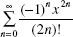# AP Calculus BC Practice Test 25

### Test Information8 questions24 minutes

Calculator Allowed

1. You wish to estimate ex, over the interval | x | < 2, with an error less than 0.001. The Lagrange error term suggests that you usea Taylor polynomial at 0 with degree at least

2. Find the volume of the solid formed when one arch of the cycloid defined parametricallyby x = θ - sin θ, y = 1 - cos θ is rotated around the x-axis.

3. Which definite integral represents the length of the first quadrant arc of the curvedefined by x(t) = et, y(t) = 1 - t2?

4. For which function isthe Taylor series about 0?

5. The hypotenuse AB of a right triangle ABC is 5 feet, and one leg, AC, is decreasing at the rate of 2 feet per second. The rate, in square feet per second,at which the area is changing when AC = 3 is

6. At how many points on the interval [0,π] does f (x) = 2 sin x + sin 4x satisfy the Mean Value Theorem?

7. Which one of the following series converges?

8. The rate at which a purification process can remove contaminants from a tank of wateris proportional to the amount of contaminant remaining. If 20% of the contaminantcan be removed during the first minute of the process and 98% must be removed to makethe water safe, approximately how long will the decontamination process take?